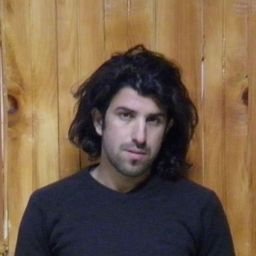Our Discord hit 10K members! 🎉 Meet students and ask top educators your questions.Join Here!Numerade Educator

# Three point charges are located at the corners of an cquilateral triangle as in Figure $\mathrm{P} 15.13$ . Find the magnitude and direction of the net electric force on the 2.00$\mu \mathrm{C}$ charge.

## See work for solution.

### Discussion

You must be signed in to discuss.
##### Top Physics 102 Electricity and Magnetism Educators
LB##### Jared E.

University of Winnipeg### Video Transcript

we have three point charges at the at the corners off any collector triangle. Oh, here is XX is here Is the y axis opposed to point Charge off seven Micro column is here. We level this point. C ah, positive to Micro Coolum charge is that Ah, this point level it A which is its origin and ah, negative four biker Coolum Charge is located at point B. Here the angle is 60 degrees. This is equipped to trying also this angle with 60 This will be 60. So we are asked to find what is the magnitude and direction off the net force Net force on there to micro Coolum Charge, which is located at point A at the origin we can find this force Uh, by drawing a forced I ground for the force F B in a so f b in a force will be attractive because this particle it eh Ah, it's pastor in this particle. It bees in 80 so they will attract each other s so we can write the force will be. It was due to Micro Coolum. The force will be in this direction which we call it f b A and the force between the sea and a will be impulsive because both charges are positive. So the direction of force will be in this direction. And if we draw, it wasn't a line here. And we see the whole angle here, Eyes 90. So this thing was 16. So this and you will be a 30 piece. And, um, we can draw this force here, which will be the compliment off this force. And this force will be again, a compliment off compliment off this force. So this force we have here is Force C A. Um So the sum of forces some of forces in X direction will be some of forcing an exertion will be this force, plus the compliment off this force, which is FC a sine theta. So this force is simply whole, um, constant to the productive charges it be in a which is a cube, be to a divided by distance, which is 0.5 meters of 0.5 meter here and 0.5 meters. Since this is a collector triangle. So is there a 0.5 square minus of this force component, which is OK, que si que es? Ah signed ADA divided by 0.5 square. Oh, this will be f c a sign 60 and this will be This will be if see a cause 30. Sorry, I said 60 year, then the total force in FX direction we get here is 3.6 times front of power minus two. Similarly, for some of forces in F y, direction will be only the force. So we have only force here. This only this compliment force we have here. So this forces to see a that this force is B A. So this component of force is simply cake. You'll see you a divided by 0.5 square distance times. Of course 30 since the direction is upward. So who level it? A minus zero point for 36 Newton after some students value for to CNN Curious So some of forces in F Y direction we got is a minus 0.46 in some off forces in F X direction. We got his 3.6 times 10 to the power minus two new too. Then we can easily find the result in force acting on two micro fool in charge, that is our is a cold square root off forces in X square plus forces in why square This gives us a resultant force off there a 0.438 You did so the magnitude of force acting on their two micro polo charged at the point, eh will be, ah 0.438 Newton. We confined angle. Also, the angle will be attaining worse off minus 0.436 divided by 3.6 times center about minus two. This gives us an angle off that our trees and minus 85.2 this is below below Positive XX is so the magnitude of force we have here is ah reporting for 38 Newton and the direction is AA minus 85.2 degree below the positive X axis.Wesleyan University
##### Top Physics 102 Electricity and Magnetism Educators
LB##### Jared E.

University of Winnipeg